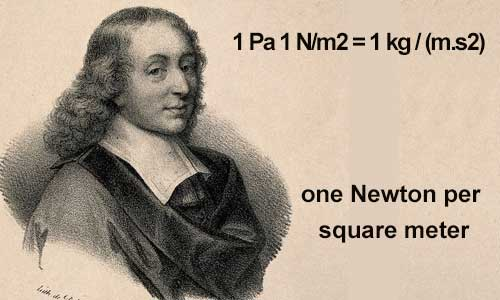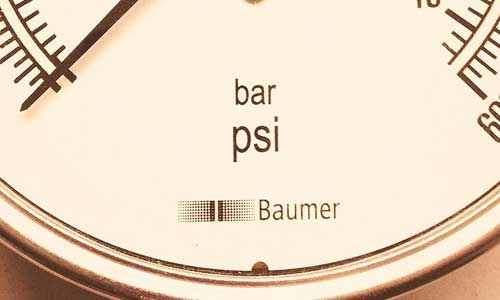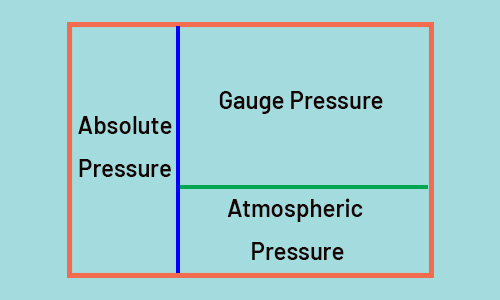# Pressure [Explanation, Pressure Units, Types of Pressure (Static, Dynamic, Stagnation)]

One of the most important quantities in industrial processes, especially those dealing with fluids, is pressure. Measuring and controlling this quantity is necessary to achieve the expected results in many industrial processes. Pressure is defined as the amount of physical and perpendicular force acting on a surface per unit area over which that force is distributed, or can also be described as the ratio of the force to the area.

Pressure can be found on surfaces, gasses, and liquids. It is calculated as follows:

P= F/A

where P is pressure

F is the normal force

and A is defined as the area of the surface.## Pressure Units

A unit is needed to express the value of each quantity, so a specific unit must be specified for the measurement of pressure.

Pressure is stated in different units. So there are different types of pressure units or scales to describe the pressure that is used depending on the country, industry, and application some of which we will discuss in this article.

### Pascal

As was discussed In the SI system, the standard unit for pressure is the  Pascal (Pa), which is equal to one Newton per square meter.

The Pascal (Pa) in the SI unit of pressure is equal to one newton per square meter.

• 1 Pa 1 N/m2 = 1 kg / (m.s2)

Pascal is used for measuring low air pressures, in applications such as measuring airflow through ventilation ducts on HVAC or building management systems.### Pounds per square inch – psi

In the English system, the standard unit for measuring pressure is the  pound per square inch (psi). It is defined as a force of one pound force applied to an area of one square inch.

• 1 psi 1 lbf/in2 = 4.45 N / (0.0254 m)2 ≈ 6895 kg/m2### Bar

Bar is one of the most popular pressure units, particularly in European countries. It is not an SI unit and commonly used in the industry and in meteorology.

A barometer is a device used in meteorology to measure atmospheric pressure. One bar is equal to 100 000 Pa and is often used to represent atmospheric pressure.

Millibar as a small value used for meteorological purposes and low range gas pressures is taken directly from the bar pressure unit and is equal to 1,000 mbar. In the SI unit of pressure, 1 mbar equals 100 Pascal.

 PSI BAR 1 0.07 2 0.14 3 0.21 5 0.34 10 0.69 20 1.38 50 3.45 100 6.89 1000 68.95

The bar unit is the most popular unit in pressure measurement. I recommend you to click on the link to find the  pressure sensor you need with the bar measuring unit.

### Standard Atmospheres (atm)

The unit atmosphere ( atm) is about equal to the atmospheric pressure and is defined as 101,325 Pa. when the reference to the real atmosphere is important in scientific fields, Atmospheres can be used.

### torr (Torr)

For measuring very small pressures, the unit torr can be useful that is almost equal to 1 mm of Hg and 1 atm is exactly equal to 760 Torr

### Manometric Units

Manometric units such as  millimeters of mercury, centimeters of mercury (cmHg), inches of mercury (inHg or “Hg), millimeters of water (mmH2O), centimeters of water (cmH2O), and inches of water (inH2O, “H2O, or iwc) are units that are used to express pressures using the change in height change of a liquid and also refer to a liquid affected by air pressure.

In the SI unit of pressure, 1 mmHg = 133.3 Pa, and atmospheric pressure is equal to 760 mmHg. Manometric units change from location to location, depending on the density and temperature of the liquid.

All pressure units have a value defined in pascals and are directly proportional to each other. Pressure values can be defined using whole units with a unique name (e.g. bar or kPa).

#### PSIA

The unit of measurement of PSIA is based on the English system and is equivalent to pounds per square inch. It indicates that the reference for measuring pressure is the absolute vacuum.

The pressure expressed in psia indicates the atmospheric air pressure + the pressure created. Atmospheric air pressure at sea level is about 14.7 psi but the pressure of a vessel completely void of any air molecules is 0 PSIA.

#### PSIG

PSIG stands for Pounds per square in gauge and refers to the gauge pressure. PSIG is a pressure measurement relative to the ambient pressure or atmospheric pressure.

A vessel that is complete without any air molecules (at sea level) is equal to -14.7 PSIG, and ambient air pressure is always measured as 0 PSIG. This unit specifies the difference between the pressure in a pipe or tank and the pressure of the atmosphere (atm).

#### barD

Bard is the unit for measuring differential pressure.

#### Barg

Barg is the pressure measurement unit for the measurement of gauge pressure. Gauge pressure is measured relative to current ambient pressure. It means absolute pressure minus atmospheric pressure.

#### Bara

It is a unit of pressure that is measured relative to an absolute vacuum. Bara means barg + atmospheric pressure (Atm).

Types of Pressure

### Static Pressure

Static pressure, sometimes called hydrostatic pressure, is the pressure exerted by a fluid at rest, or the pressure at a point in a fluid is called static pressure and Its value is independent of direction.

This fluid is often liquid or gas. Since the fluid does not move, static pressure is the result of the weight of the fluid or the gravitational force exerted on the fluid particles.### Dynamic Pressure

Dynamic pressure or velocity pressure is the pressure caused by the movement of a fluid. Forces must be applied to move objects, so the moving objects have kinetic energy.

Now, if there is an obstacle in the way of this object or fluid, the force from the kinetic energy will be transferred to the obstacle in the direction of motion and will push it.

This type of pressure, which depends on the direction of motion, is called dynamic pressure. Dynamic pressure is equal to the difference between stagnation pressure and static pressure. It is especially important in flowing fluids.

### Stagnation Pressure

Stagnation pressure (pitot pressure or total pressure) is the static pressure at the stagnation point in the flow of a fluid. At the point of stagnation, the flow comes to rest and the velocity of the fluid decelerates to zero speed and all kinetic energy is converted to pressure isotropically.

It is defined as the maximum pressure experienced by the fluid when the fluid is brought to rest.

All you read here was just an introduction. In another article, we'll talk about different types of pressure, such as absolute, gauge, etc. If you're in need of a pressure sensor, you can check the link to view them. There are also lots of other articles about pressure and pressure sensor. Here are three of them, in case you like to know more:

12th Apr 2022 Saeed Abd

• ## From Lab to Industry: Pressure Sensing Diaphragm at Work

In a world pulsing with technology and innovation, there exists an unsung hero—often humble in ap …
2nd Dec 2023
• ## Transforming Noise into Knowledge: The Art of Signal Conditioning

Signal conditioning circuitry is the unsung hero of modern electronics, silently working behind t …
29th Nov 2023
• ## Beyond the Surface: The Inner Workings of a Pressure Transmitter

Imagine a world where the unseen forces of pressure are harnessed, measured, and transformed into …
27th Nov 2023